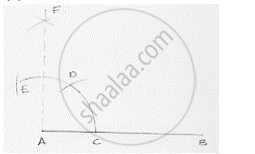# Construct the Following Angles at the Initial Point of a Given Ray and Justify the Construction 90°. - Mathematics

Construct the following angles at the initial point of a given ray and justify the construction 90°.

#### SolutionSteps of construction:
1. Draw a line segment AB.
2. With center A and any radius draw in arc which intersect AB at C.
3. With center C and same radius draw an arc which intersects previous arc at D.
4. With centers D same radius draw an arc which intersects are in (2) at E.
5. With centers E and D same radius more than 1/2ED draw an arc cutting each other at F.
6. Join FA
∴ ∠FAB = 90°

Concept: Basic Constructions
Is there an error in this question or solution?

#### APPEARS IN

RD Sharma Mathematics for Class 9
Chapter 16 Constructions
Exercise 16.2 | Q 10.2 | Page 9

Share